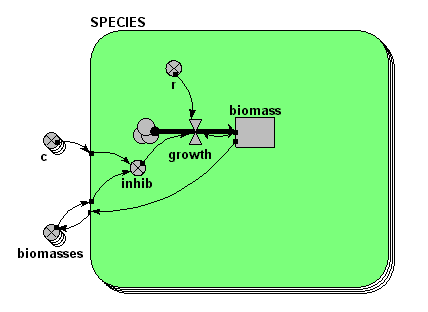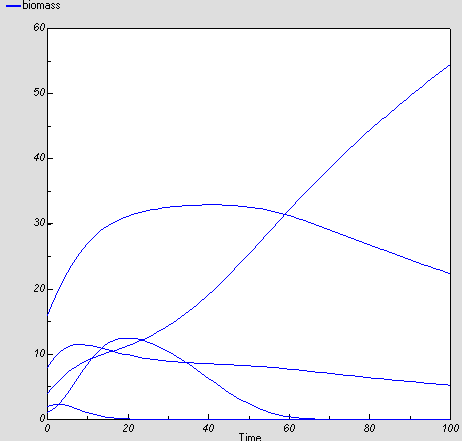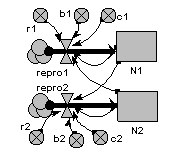# Competition

## Multi-species competition model

ModelId:
grace1
SimileVersion:
3.1+

This is a multi-species version of the classic Lotka-Volterra competition model. The dynamics of each species is given by:

dBi/dt = ri*(1-sumj = 1,nsp(ci,j*Bj))

where:
Bi is the biomas of species i;
ri is the maximum specific growth rate for species i;Results:Model tags:

## Lotka-Volterra two-species competition model

ModelId:
competition1
SimileVersion:
3.1+This is the classic textbook model of the population dynamics of two competing species.

It is based on a pair of differential equations, each having the same mathematical form:

dX1/dt = r1.X1.(1-b1.X1-c1.X2)
dX2/dt = r2.X2.(1-b2.X2-c2.X1)

Results: